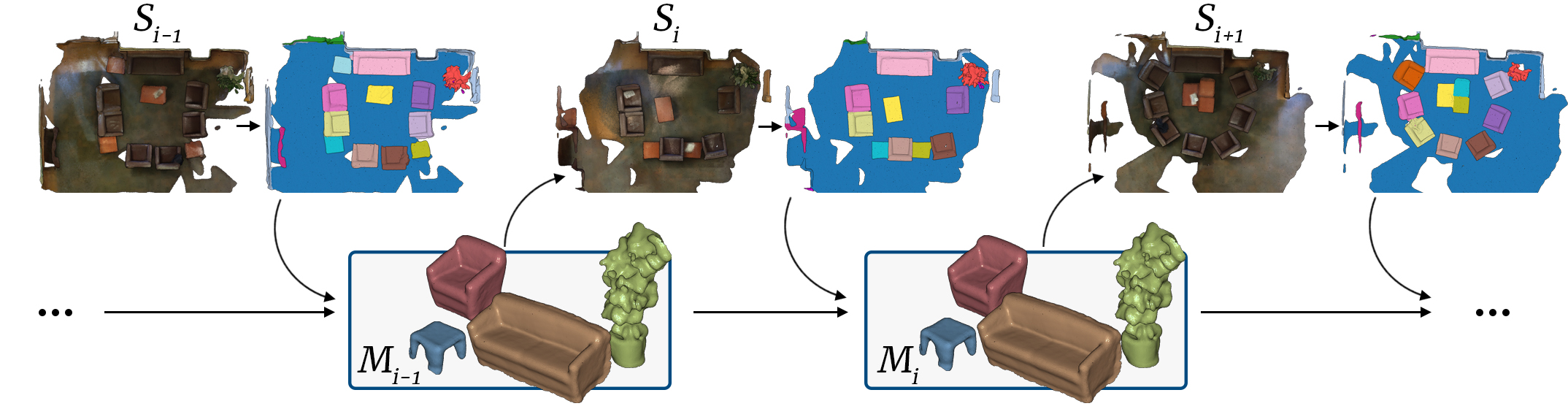Rescan: Inductive Instance Segmentation for Indoor RGBD Scans

IEEE International Conference on Computer Vision (ICCV) 2019The proposed method estimates a persistent, temporally-aware scene model $$M_i$$ from a series of scene observations $$S_i$$, captured at sparse time intervals. $$M_{i-1}$$ is used to estimate an arrangement of objects in each novel observation $$S_i$$. The estimated arrangement is used to estimate the instance segmentation of $$S_i$$, which is then used to update the model $$M_i$$.

Abstract

In depth-sensing applications ranging from home robotics to AR/VR, it will be common to acquire 3D scans of interior spaces repeatedly at sparse time intervals (e.g., as part of regular daily use). We propose an algorithm that analyzes these rescans'' to infer a temporal model of a scene with semantic instance information. Our algorithm operates inductively by using the temporal model resulting from past observations to infer an instance segmentation of a new scan, which is then used to update the temporal model. The model contains object instance associations across time and thus can be used to track individual objects, even though there are only sparse observations. During experiments with a new benchmark for the new task, our algorithm outperforms alternate approaches based on state-of-the-art networks for semantic instance segmentation.

Citation

@article{HalberRescan19,
title     = {Rescan: Inductive Instance Segmentation for Indoor RGBD Scans},
author    = {Maciej Halber and Yifei Shi and Kevin Kai Xu and Thomas Funkhouser},
booktitle = {ICCV},
year      = {2019}
}

Data

The Rescan Dataset contains 3D reconstructions of 45 sequences of 13 distinct common spaces like lounges, study areas and living rooms. Each space has been captured between 3 to 5 times. Between each capture, the objects within the scene were moved in the way that long-term changes that are likely to occur in such spaces. In most cases, objects were newly introduced or removed from the scene as they would be in natural use. The captured spaces are relatively large, with an average approximate area of $$67.58m^2$$.

The main feature of the Rescan dataset is the presence of object associations across time. These associations are expressed in the form of stable instance segmentation -- object A has the same unique instance id in all time-steps $$t_0, t_1, ..., t_n$$.

Folder Structure

rescan_dataset_root
├── scene_a
|   └── color [color video streams for each sequence]
|   └── depth [depth video streams for each sequence]
|   └── gt_segmentation [ply files containing seqmentation]
├── scene_b
├── ...
└── scene_m

Ply File Format

All Rescan Ply files are stored in binary little endian format. Apart from standard $$[x,y,z]$$, $$[nx, ny, nz]$$, $$[red, green, blue]$$ flags for position, normal and color, this format also stores $$radius$$ property for visualization, $$class\_idx$$ for semantic class id, and $$instance\_idx$$ for instance id. Overall the ply header is:

ply
format binary_little_endian 1.0
element vertex 282892
property float x
property float y
property float z
property float nx
property float ny
property float nz
property uchar red
property uchar green
property uchar blue